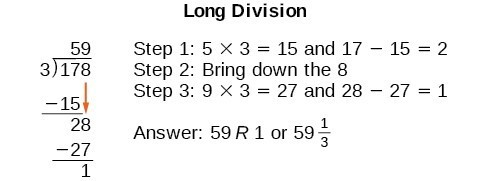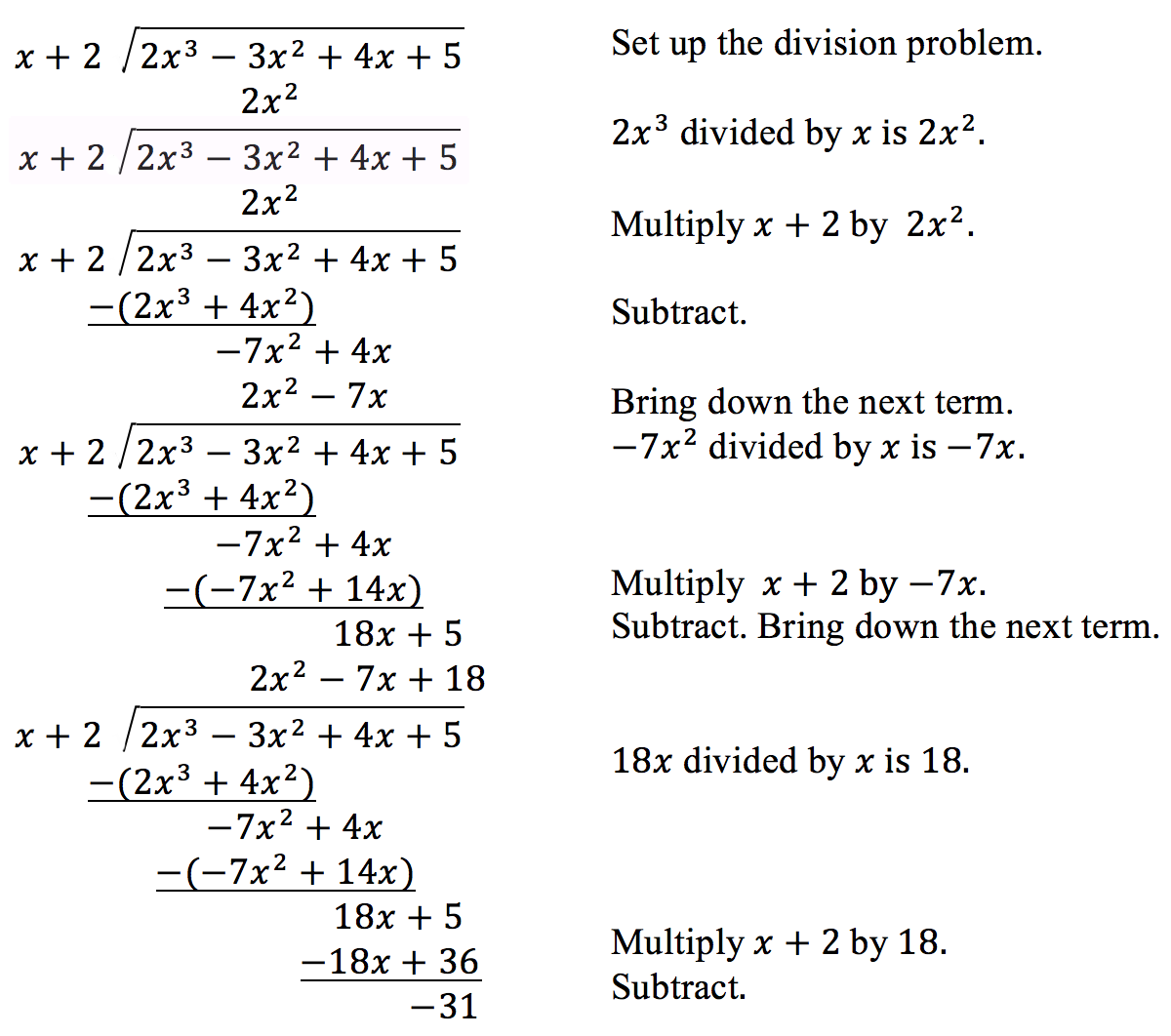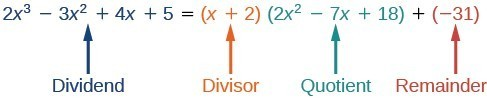## 8.3 – Division of Polynomials

### Learning Objectives

• (8.3.1) – Divide a polynomial by a monomial
• (8.3.2) – Perform long division of polynomials
• (8.3.3) – Applications of polynomial division

The exterior of the Lincoln Memorial in Washington, D.C., is a large rectangular solid with length 61.5 meters (m), width 40 m, and height 30 m. We can easily find the volume using elementary geometry.

$\begin{array}{l}V=l\cdot w\cdot h\hfill \\ \text{ }=61.5\cdot 40\cdot 30\hfill \\ \text{ }=73,800\hfill \end{array}$

So the volume is 73,800 cubic meters $\left(\text{m}{^3} \right)$. Suppose we knew the volume, length, and width. We could divide to find the height.

$\large \begin{array}{l}h=\frac{V}{l\cdot w}\hfill \\ \text{ }=\frac{73,800}{61.5\cdot 40}\hfill \\ \text{ }=30\hfill \end{array}$

As we can confirm from the dimensions above, the height is 30 m. We can use similar methods to find any of the missing dimensions. We can also use the same method if any or all of the measurements contain variable expressions. For example, suppose the volume of a rectangular solid is given by the polynomial $3{x}^{4}-3{x}^{3}-33{x}^{2}+54x$. The length of the solid is given by $3x$; the width is given by $x - 2$. To find the height of the solid, we can use polynomial division, which is the focus of this section.Lincoln Memorial, Washington, D.C. (credit: Ron Cogswell, Flickr)

# (8.3.1) – Divide a polynomial by a monomial

Division of polynomials isn’t much different from division of numbers. In the exponential section, you were asked to simplify expressions such as: $\displaystyle\frac{{{a}^{2}}{{({{a}^{5}})}^{3}}}{8{{a}^{8}}}$. This expression is the division of two monomials. To simplify it, we divided the coefficients and then divided the variables. In this section we will add another layer to this idea by dividing polynomials by monomials, and by binomials.

The distributive property states that you can distribute a factor that is being multiplied by a sum or difference, and likewise you can distribute a divisor that is being divided into a sum or difference. In this example, you can add all the terms in the numerator, then divide by 2.

$\displaystyle \frac{\text{dividend}\rightarrow}{\text{divisor}\rightarrow}\,\,\,\,\,\, \frac{8+4+10}{2}=\frac{22}{2}=11$

Or you can first divide each term by 2, then simplify the result.

$\displaystyle \frac{8}{2}+\frac{4}{2}+\frac{10}{2}=4+2+5=11$

Either way gives you the same result. The second way is helpful when you can’t combine like terms in the numerator.  Let’s try something similar with a binomial.

### Example

Divide. $\displaystyle \frac{9a^3+6a}{3a^2}$

In the next example, you will see that the same ideas apply when you are dividing a trinomial by a monomial. You can distribute the divisor to each term in the trinomial and simplify using the rules for exponents. As we have throughout the course, simplifying with exponents includes rewriting negative exponents as positive. Pay attention to the signs of the terms in the next example, we will divide by a negative monomial.

### Example

Divide. $\displaystyle \frac{27{{y}^{4}}+6{{y}^{2}}-18}{-6y}$

The same principle will apply for polynomials with more than one variable.

### ExAMPLE

Divide. $\displaystyle \frac{18x^3y-36xy^2+12x}{-3xy}$

Notice, that in the examples above, the answer was a rational expression.

# (8.3.2) – Performing Long Division of Polynomials

We are familiar with the long division algorithm for ordinary arithmetic. We begin by dividing into the digits of the dividend that have the greatest place value. We divide, multiply, subtract, include the digit in the next place value position, and repeat. For example, let’s divide 178 by 3 using long division.Another way to look at the solution is as a sum of parts. This should look familiar, since it is the same method used to check division in elementary arithmetic.

$\begin{array}{l}\text{dividend = }\left(\text{divisor }\cdot \text{ quotient}\right)\text{ + remainder}\hfill \\ 178=\left(3\cdot 59\right)+1\hfill \\ =177+1\hfill \\ =178\hfill \end{array}$

We call this the Division Algorithm and will discuss it more formally after looking at an example.

Division of polynomials that contain more than one term has similarities to long division of whole numbers. We can write a polynomial dividend as the product of the divisor and the quotient added to the remainder. The terms of the polynomial division correspond to the digits (and place values) of the whole number division. This method allows us to divide two polynomials. For example, if we were to divide $2{x}^{3}-3{x}^{2}+4x+5$ by $x+2$ using the long division algorithm, it would look like this:We have found

$\displaystyle \frac{2{x}^{3}-3{x}^{2}+4x+5}{x+2}=2{x}^{2}-7x+18-\frac{31}{x+2}$

or

$2{x}^{3}-3{x}^{2}+4x+5=\left(x+2\right)\left(2{x}^{2}-7x+18\right)-31$

We can identify the dividend, the divisor, the quotient, and the remainder.Writing the result in this manner illustrates the Division Algorithm.

### A General Note: The Division Algorithm

The Division Algorithm states that, given a polynomial dividend $f\left(x\right)$ and a non-zero polynomial divisor $d\left(x\right)$ where the degree of $d\left(x\right)$ is less than or equal to the degree of $f\left(x\right)$, there exist unique polynomials $q\left(x\right)$ and $r\left(x\right)$ such that

$f\left(x\right)=d\left(x\right)q\left(x\right)+r\left(x\right)$

$q\left(x\right)$ is the quotient and $r\left(x\right)$ is the remainder. The remainder is either equal to zero or has degree strictly less than $d\left(x\right)$.

If $r\left(x\right)=0$, then $d\left(x\right)$ divides evenly into $f\left(x\right)$. This means that, in this case, both $d\left(x\right)$ and $q\left(x\right)$ are factors of $f\left(x\right)$.

### How To: Given a polynomial and a binomial, use long division to divide the polynomial by the binomial.

1. Set up the division problem.
2. Determine the first term of the quotient by dividing the leading term of the dividend by the leading term of the divisor.
3. Multiply the answer by the divisor and write it below the like terms of the dividend.
4. Subtract the bottom binomial from the top binomial.
5. Bring down the next term of the dividend.
6. Repeat steps 2–5 until reaching the last term of the dividend.
7. If the remainder is non-zero, express as a fraction using the divisor as the denominator.

### Example: Using Long Division to Divide a Second-Degree Polynomial

Divide $5{x}^{2}+3x - 2$ by $x+1$.

### Example: Using Long Division to Divide a Third-Degree Polynomial

Divide $6{x}^{3}+11{x}^{2}-31x+15$ by $3x - 2$.

### EXAMPLE

Divide $16{x}^{3}-12{x}^{2}+20x - 3$ by $4x+5$.

### Example

Divide: $\displaystyle \frac{\left(x^{3}–6x–10\right)}{\left(x–3\right)}$

In the video that follows, we show another example of dividing a degree three trinomial by a binomial, also with a “missing” term..

# (8.3.3) – Application of Polynomial Division

Polynomial division can be used to solve a variety of application problems involving expressions for area and volume. We looked at an application at the beginning of this section. Now we will solve that problem in the following example.

### Example: Using Polynomial Division in an Application Problem

The volume of a rectangular solid is given by the polynomial $3{x}^{4}-3{x}^{3}-33{x}^{2}+54x$. The length of the solid is given by $3x$ and the width is given by $x-2$. Find the height of the solid.

### ExAMPLE

The area of a rectangle is given by $3{x}^{3}+14{x}^{2}-23x+6$. The width of the rectangle is given by $x+6$. Find an expression for the length of the rectangle.

1. National Park Service. "Lincoln Memorial Building Statistics." http://www.nps.gov/linc/historyculture/lincoln-memorial-building-statistics.htm. Accessed 4/3/2014